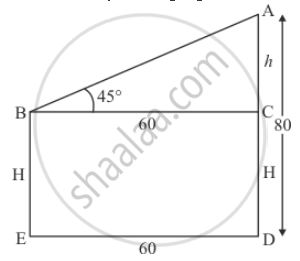# The Horizontal Distance Between Two Trees of Different Heights is 60 M. the Angle of Depression of the Top of the First Tree, When Seen from the Top of the Second Tree, is 45°. If the Height of the Second Tree is 80 M, Find the Height of the First Tree. - Mathematics

The horizontal distance between two trees of different heights is 60 m. The angle of depression of the top of the first tree, when seen from the top of the second tree, is 45°. If the height of the second tree is 80 m, find the height of the first tree.

#### Solution

Let the difference between two trees be DE = 60 m and angle of depression of the first tree from the top to the top of the second tree is ∠ABC = 45°.

Let BE = H m, AC = h m, AD = 80m.

We have to find the height of the first tree

The corresponding figure is as followsIn ΔABC

=> tan B = (AC)/(BC)

=> tan 45° = h/60

=> 1 - h/60

=> h = 60

Since H = 80 - h

=> H = 80 - 60

=> H = 20

Hence the heght of first tree is 20 m

Concept: Heights and Distances
Is there an error in this question or solution?

#### APPEARS IN

RD Sharma Class 10 Maths
Chapter 12 Trigonometry
Exercise 12.1 | Q 47 | Page 32